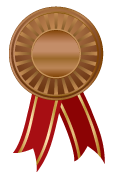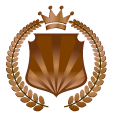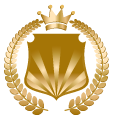## Student Console## General AwardsBronze Medal Each score of 100% on a Basic or Advanced Math chapter quiz.
Each score of 70-79% on a course final quizSilver Medal Each score of 100% on a Pre-Algebra chapter quizGold Medal Each score of 100% on a Algebra chapter quiz

## Premiere AwardsBronze cup Earn in each course by scoring 70-79% on a course final quizSilver Cup Earn in each course by scoring 80-89% on a course final quizGold Cup Earn in each course by scoring 100% on a course final quiz

## Ultimate AwardsThe Bronze Medallion Earn in each course by scoring 100% on at least 5 chapter quizzesThe Silver Medallion Earn in each course by scoring 100% on at least 10 chapter quizzesThe Gold Medallion Earn in each course by scoring 100% on the course final quiz and completing every lesson.
Scroll to TopPlease cancel my Math and Algebra subscription. I understand that my account will be cancelled and I will no longer have access as of my next renewal date.This is a Final Course Quiz. The quiz will contain a random selection of 50 problems from all of the Course Chapters covered. If you have watched the video tutorials and completed the practice exercises for all the lessons in each chapter, you should be well prepared for this quiz. If you feel you need more time to study, you can go back to any lesson and review the video and exercises. Make sure that you are always prepared with plenty of scratch paper and pencils before you begin the quiz.

The questions are selected at random from a pool of problems so the quiz will be different each time you take it.

Most answers will be in the form of multiple choice or fill in the blank, but there may be other types as well. Fill in the blank answers need to be in the correct format or the problem will be marked as incorrect.

• Make sure there are no spaces before or after you type in your answer.
• Answers that require a comma may be entered with or without the comma, but remember, no spaces.
EXAMPLE: 1280 and 1,280 are both in the correct format.
• Certain answers such as fractions will have multiple fields.
EXAMPLE:• There is a box for the whole number, numerator, and denominator. If any of the boxes do not require an answer, it may be left blank. For instance, if the answer is 6, then you would enter 6 in the first box and leave the numerator, and denominator box blank. In another instance, if the answer is 3/4, then you would leave the first box blank and enter 3 in the 2nd box (numerator) and 4 in the 3rd box (denominator). An answer of 4 2/5 would require each box to be filled. A 4 in the first box, a 2 in the 2nd and a 5 in the 3rd for the answer of four and two fifths.

You will have 2 hours to complete this quiz, and must score a 70% to pass. If there are 50 questions in this quiz, how many correct answers will you need to pass?

Good Luck!Course 1

Basic Math

## Course Description

Basic Math is the foundation for all the other courses. It provides a solid foundation for all the necessary skills development. The student is introduced to a variety of terms, procedures and methodology that will prepare them for the more advanced courses.

This is a must for all 4th and 5th graders. It will form the foundation for success in all future math classes: Whole Numbers, Fractions, Decimals, Percents, Integers, Geometry, Charts and Graphs, Problem Solving, Ratios and Proportions, Probability and Statistics, Number Theory, Algebra, Graphing on Number Lines and Coordinate Systems.

## Basic Math ChaptersCourse 2

## Course Description

Advanced Math provides a much deeper understanding of mathematical concepts. It builds upon what was learned in Basic Math and starts laying the groundwork for Algebra.

This course has many of the topics from Basic Math but goes into much more depth. Students will be equipped with the math tools to meet all of the challenges of Whole Numbers, Fractions, Decimals, Percents, Integers, Geometry, Charts and Graphs, Problem Solving, and much more, while preparing for Pre-Algebra and Algebra.Course 3

Pre-Algebra

## Course Description

Students are now ready for Algebra after the preparation and skill sets learned in the previous three courses. This is the longest of the four course curriculum and covers all algebraic functions and methods.

This course is a complete algebra program that explains each topic in a way that is easy to understand. Short, concise, self-contained lessons. Lots of examples with fully explained step-by-step solutions. Review is built into each lesson to ensure mastery. Lesson quizzes and a final quiz allow students to measure success.

## Pre-Algebra ChaptersAlgebra Course 4

## Course Description

Basic Math is the foundation for all the other courses. It provides a solid foundation for all the necessary skills development. The student is introduced to a variety of terms, procedures and methodology that will prepare them for the more advanced courses.

This is a must for all 4th and 5th graders. It will form the foundation for success in all future math classes: Whole Numbers, Fractions, Decimals, Percents, Integers, Geometry, Charts and Graphs, Problem Solving, Ratios and Proportions, Probability and Statistics, Number Theory, Algebra, Graphing on Number Lines and Coordinate Systems.

## Algebra Chapters## Thank You!

We appreciate you reaching out to us. Someone will get back to your within 24 hours.

## This website uses cookies for marketing analytics. We do not share or sell data to any third party. All data collected is to ensure you get the best experience on our website. By remaining on our website and closing this box, you agree to our policy.This is a Chapter Quiz. The quiz will contain a random selection of problems from all of the lessons covered. If you have watched the video tutorials and completed the practice exercises for all the lessons, you should be well prepared for this quiz. If you feel you need more time to study, you can go back to any lesson and review the video and exercises. Make sure that you are always prepared with plenty of scratch paper and pencils before you begin the quiz.

You can return and take this quiz as many times as you like. The questions are selected at random from a pool of problems so the quiz will be different each time. It is recommended that you take the quiz several times until you can answer each questions correctly.

Most answers will be in the form of multiple choice or fill in the blank, but there may be other types as well. Fill in the blank answers need to be in the correct format or the problem will be marked as incorrect.

• Make sure there are no spaces before or after you type in your answer.
• Answers that require a comma may be entered with or without the comma, but remember, no spaces.
EXAMPLE: 1280 and 1,280 are both in the correct format.
• Certain answers such as fractions will have multiple fields.
EXAMPLE:• There is a box for the whole number, numerator, and denominator. If any of the boxes do not require an answer, it may be left blank. For instance, if the answer is 6, then you would enter 6 in the first box and leave the numerator, and denominator box blank. In another instance, if the answer is 3/4, then you would leave the first box blank and enter 3 in the 2nd box (numerator) and 4 in the 3rd box (denominator). An answer of 4 2/5 would require each box to be filled. A 4 in the first box, a 2 in the 2nd and a 5 in the 3rd for the answer of four and two fifths.

Good Luck!